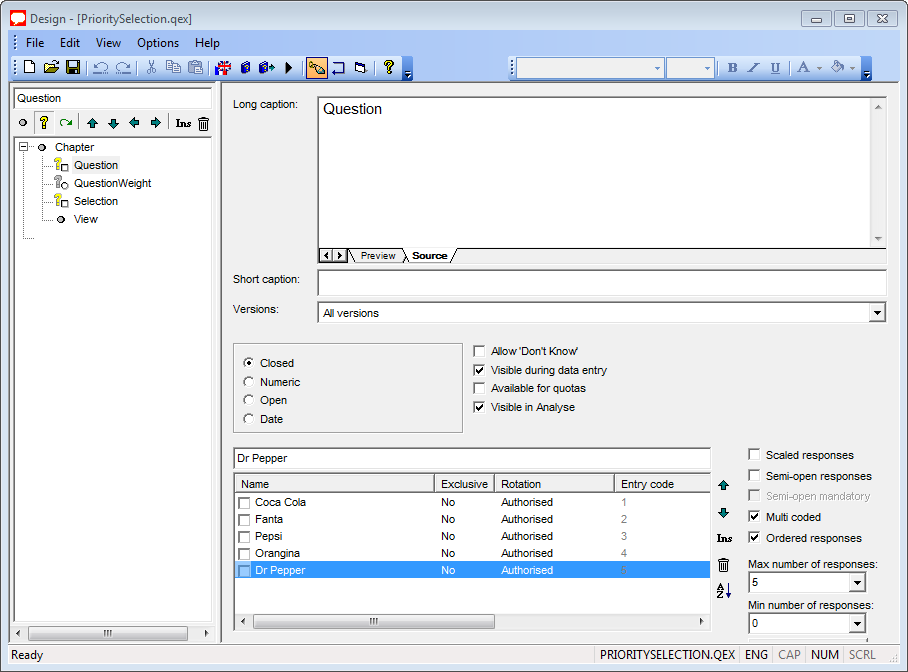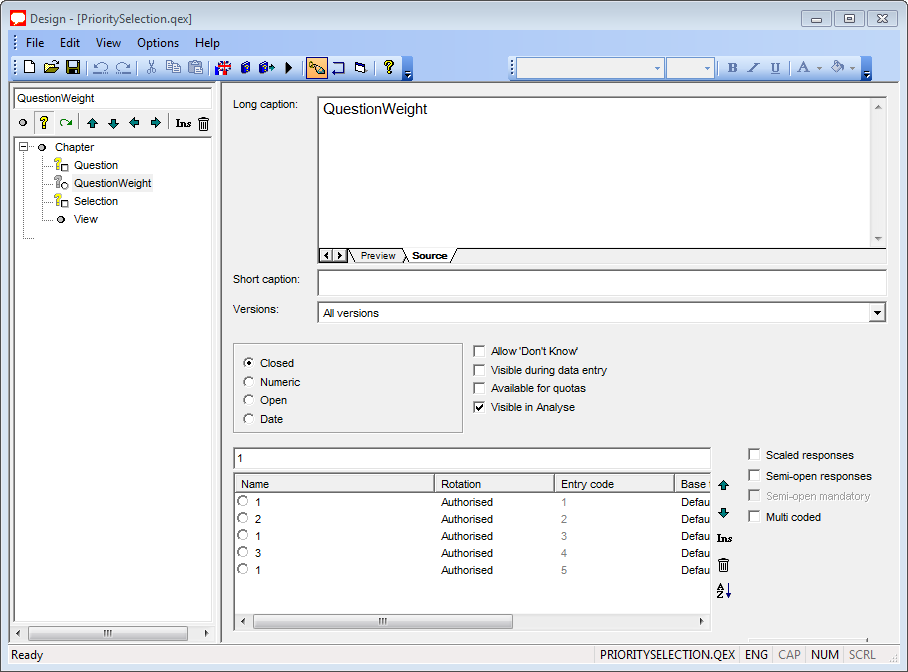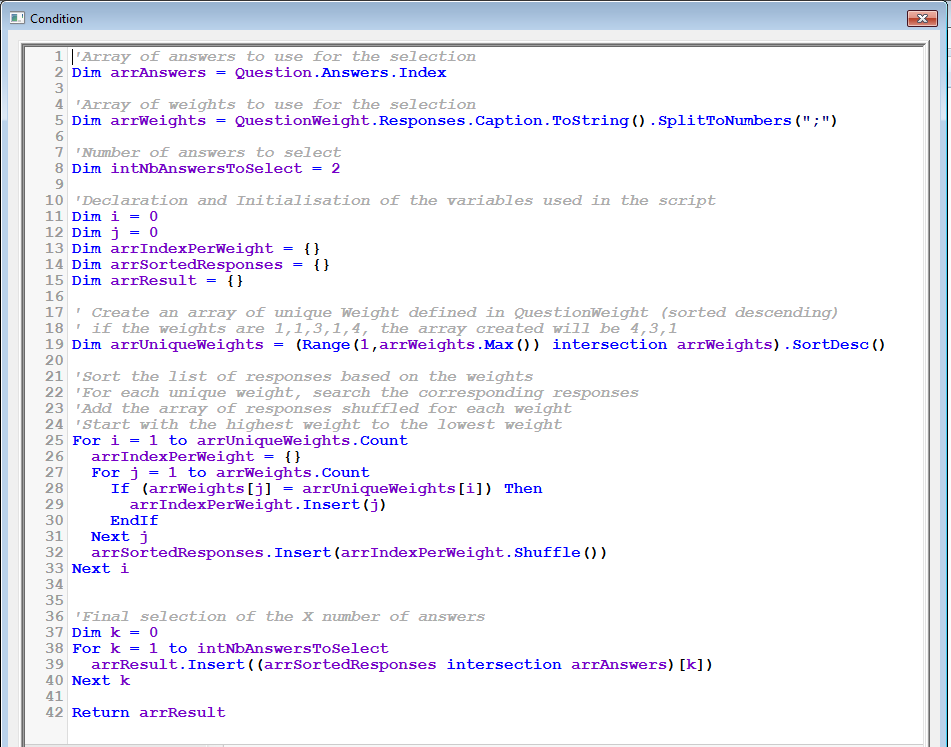Scroll

# Priority selection using askiascript 2.0

Follow

Here we would like to select X number of responses from a multiple closed question based on priority for each of them and using askiascript 2.0.

Before starting to detail the example, we will explain two notions of the askiascript which will be used for the selection.

1. Intersection:

Intersection retrieves the common values of two sets. It compares the selected responses given to two questions, and discovers which responses were selected in both. For the value to be present in the intersection of two sets, it must be present in both of the sets being combined. Note that the order of the returned items is determined by the leftmost set (the one before the intersection keyword).

So if you write:

`Q1 intersection {1;3;5;7;9}`

and Q1 = {5;8;9;1;2} then the result will be {5;9;1}

and if you write:

`{1;3;5;7;9} intersection Q1`

and Q1 = {5;8;9;1;2} then the result will be {1;5;9}

2. Isolate the first or second or third... value of a set using []

To be able to isolate the second value of a set, we can write:

`{1;3;5;7;9}`

and the result will be {3}

So we can also write something like this:

`({1;3;5;7;9} intersection Q1)`

and Q1 = {5;8;9;1;2} then the result will be {5} because the set returned is {1;5;9}

Principle of the selection

We have one multiple closed question with 5 responses:

• 1. Coca Cola (1)
• 2. Fanta (2)
• 3. Pepsi (1)
• 4. Orangina (3)
• 5. Dr Pepper (1)

and we would like to select X number of responses selected from this multiple closed question but each of them have a weight. Here Orangina has the biggest priority/weight, then followed by Fanta and at last, the rest of the responses. The weight of each of them are between brackets.

The idea of the selection will be to reorder the 5 responses based on the weight given to each of them. We will also make sure to shuffle the responses with the same weight.

So here it will be

`{4;2;5;1;3}`

Then we will apply an intersection with the answers given/selected at the question:

`{4;2;5;1;3} intersection Question`

if Question = {1;3;4} then the result will be {4;1;3}

And finally we will pick X number of responses from the set returned so if we want to select 2 responses then we will pick :

`({4;2;5;1;3} intersection Question) => {4}`

and also

`({4;2;5;1;3} intersection Question) => {1}`

Details of the qex file example

First we create the multiple closed question with the list of 5 responses:Then we create a non visible question to store the weight of each responses:Finally, we create one routing set value to the variable Selection with the following script:If you want to modify/use this code, then you just have to change the list of responses in the Question and the corresponding weight in QuestionWeight and in the script, modify the line 2, 5 and 8 to refer to the good shortcut question and the good number of responses to select.

1 out of 1 found this helpful
Have more questions? Submit a request

•Amal Mohamed

I downloaded the sample file but I can't test it because there is duplicated responses for QuestionWeight!

•Jérôme Duparc

Hi Amal,

In design->options menu->settings->Allow duplicate modalities->Set it to yes.

Best Regards